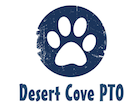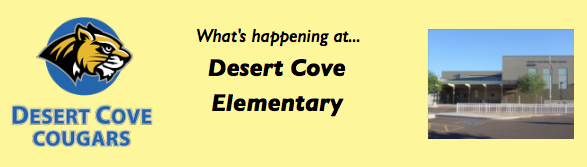/* styles */ Hi DC Community!# The Desert Cove eNews is sent on a MONTHLY basis.JANUARY

▪ Jan 15: NO SCHOOL
▪ Jan 15: SMHS Matadors in Training Clinic
▪ Jan 17: United Parent Council Meeting
▪ Jan 23: EARLY RELEASE
▪ Jan 25: Yumberi Yogurt Restaurant Night
▪ Jan 26: Breakfast with Buddies
▪ Jan 31: Teacher Conference Dinner
 ▪ Jan 15: NO SCHOOL
 ▪ Jan 15: SMHS Matadors in Training Clinic
 ▪ Jan 17: United Parent Council Meeting
 ▪ Jan 23: EARLY RELEASE
 ▪ Jan 25: Yumberi Yogurt Restaurant Night
 ▪ Jan 26: Breakfast with Buddies
 ▪ Jan 31: Teacher Conference Dinner

 table div table+table+table+table+table+table+table div table{width:100%;padding:0}table div table+table+table+table+table+table+table div table img{width:96.23%;padding:0;float:none}table div table+table+table+table+table+table+table div table td{width:100%;padding:0 1.88% 18px}/* styles */## SMHS Matadors in Training Clinic & Basketball GameJan 15th & 16th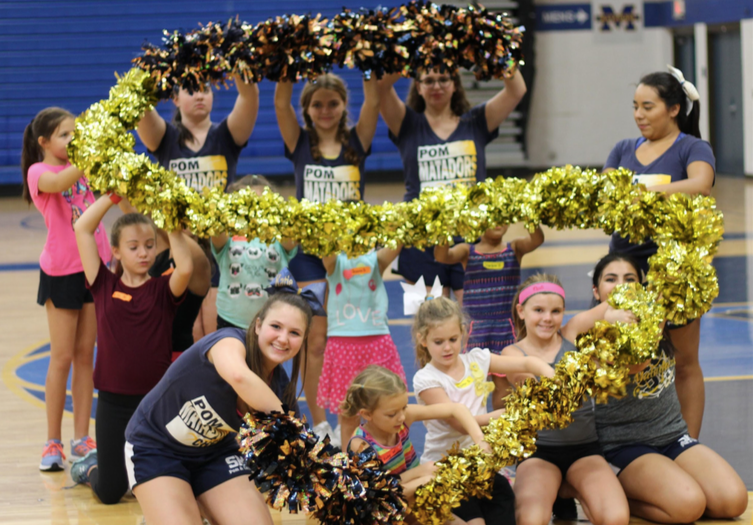table div table+table+table+table+table+table+table+table+table+table+table div table{width:100%;padding:0}table div table+table+table+table+table+table+table+table+table+table+table div table img{width:96.23%;padding:0;float:none}table div table+table+table+table+table+table+table+table+table+table+table div table td{width:100%;padding:0 1.88% 18px}/* styles */## Yumberi Yogurt Restaurant Night - Jan 25th

 table.module-12{width:76.42%;padding:0}table div table+table+table+table+table+table+table+table+table+table+table+table+table div table{width:76.42%;float:none;margin-left:auto;margin-right:auto;padding:0}table div table+table+table+table+table+table+table+table+table+table+table+table+table div table a{border:0 none;text-decoration:none}table div table+table+table+table+table+table+table+table+table+table+table+table+table div table img{width:100%!important;border:0 none;text-decoration:none}table div table+table+table+table+table+table+table+table+table+table+table+table+table div table td{width:100%;padding:0}/* styles */

 /* styles */ Desert Cove receives 20% of all purchases!
 table div table+table+table+table+table+table+table+table+table+table+table+table+table+table+table+table div table{width:100%;padding:0}table div table+table+table+table+table+table+table+table+table+table+table+table+table+table+table+table div table img{width:96.23%;padding:0;float:none}table div table+table+table+table+table+table+table+table+table+table+table+table+table+table+table+table div table td{width:100%;padding:0 1.88% 18px}/* styles */## Breakfast with Buddies - Jan 26th at 7AM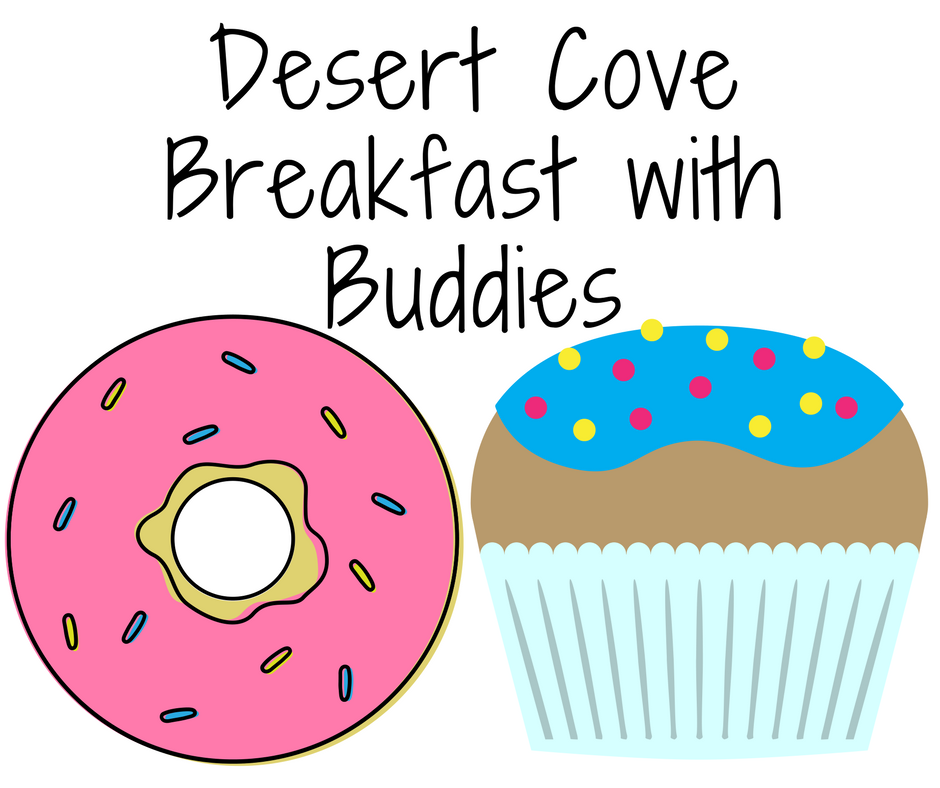/* styles */ As the needs of our Desert Cove Community change, the Events that we, as a Parent Teacher Organization coordinate, must also rise to meet those needs. It has been brought to our attention by concerned Parents, Caregivers, Teachers and Staff that many children do not attend the Muffins with Moms and Donuts with Dads events because the makeup of their family unit makes them feel as though they can not attend. The last thing we want to do is exclude any part of our Desert Cove Community, so this year it was decided to combine the two events into one BIG community event! The "Breakfast with Buddies" event will allow students to bring ANY special adult(s) in their life to enjoy this event with them! Click Here to Open the Flyer
 table div table+table+table+table+table+table+table+table+table+table+table+table+table+table+table+table+table+table+table+table div table{width:100%;padding:0}table div table+table+table+table+table+table+table+table+table+table+table+table+table+table+table+table+table+table+table+table div table img{width:96.23%;padding:0;float:none}table div table+table+table+table+table+table+table+table+table+table+table+table+table+table+table+table+table+table+table+table div table td{width:100%;padding:0 1.88% 18px}/* styles */## Angel Bin Shoe Drive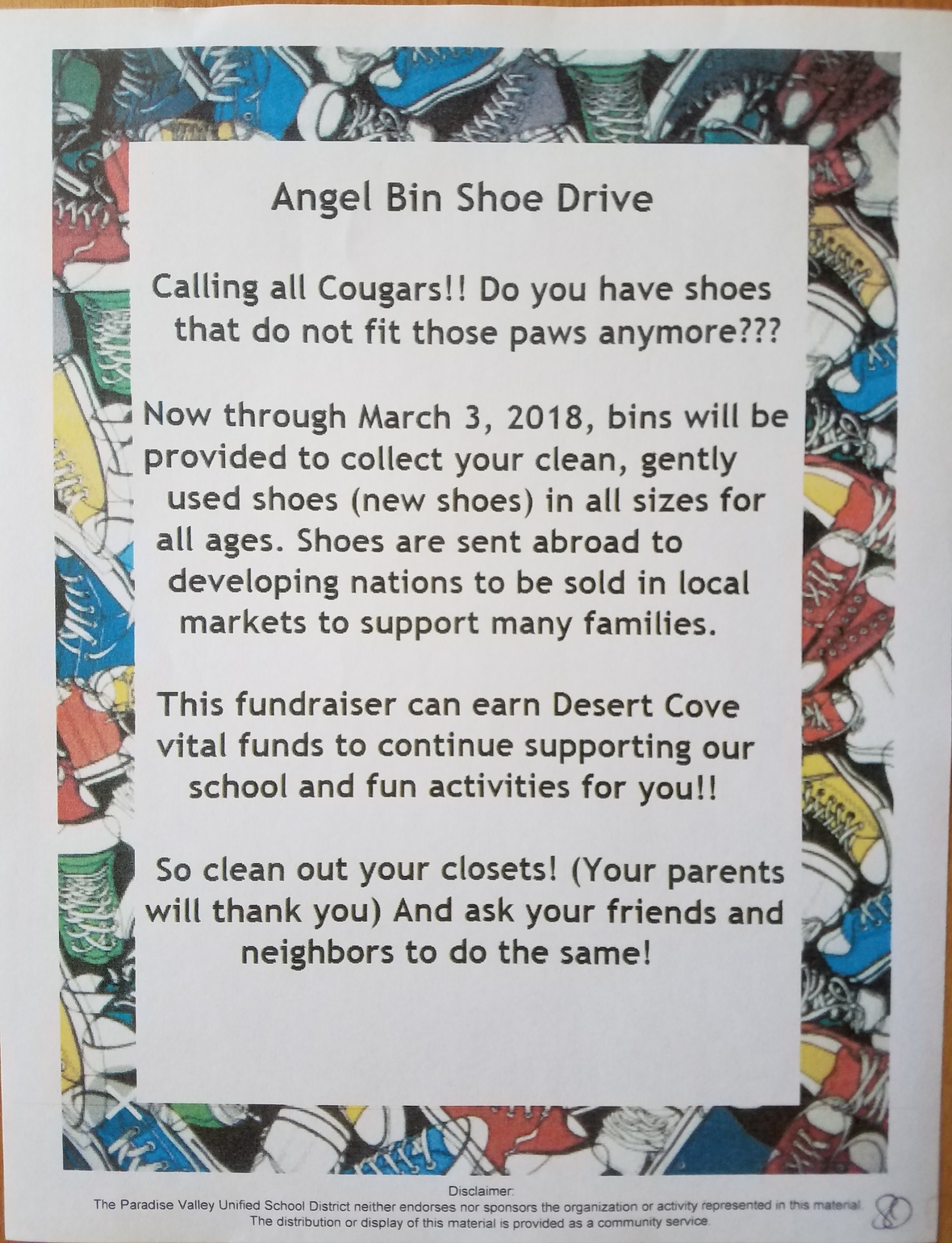table div table+table+table+table+table+table+table+table+table+table+table+table+table+table+table+table+table+table+table+table+table+table+table div table{width:100%;padding:0}table div table+table+table+table+table+table+table+table+table+table+table+table+table+table+table+table+table+table+table+table+table+table+table div table img{width:96.23%;padding:0;float:none}table div table+table+table+table+table+table+table+table+table+table+table+table+table+table+table+table+table+table+table+table+table+table+table div table td{width:100%;padding:0 1.88% 18px}/* styles */table div table+table+table+table+table+table+table+table+table+table+table+table+table+table+table+table+table+table+table+table+table+table+table+table div table td,table.module-23{width:100%;padding:0}table div table+table+table+table+table+table+table+table+table+table+table+table+table+table+table+table+table+table+table+table+table+table+table+table div table{width:100%;float:none;margin-left:auto;margin-right:auto;padding:0}table div table+table+table+table+table+table+table+table+table+table+table+table+table+table+table+table+table+table+table+table+table+table+table+table div table a{border:0 none;text-decoration:none}table div table+table+table+table+table+table+table+table+table+table+table+table+table+table+table+table+table+table+table+table+table+table+table+table div table img{width:100%!important;border:0 none;text-decoration:none}/* styles */

# All important information regarding PTO News & Events will be on the DESERT COVE PTO WEBSITE(click to open)

 /* styles */ You can also subscribe to more frequent updates through:We have created a page on the PTO website where you can access the following communications:

Desert Cove PTO eNews archives
Desert Cove Principal News
PV School District eNews
United Parent Council eNews